# Dice Reasoning: Solved examples

Q.1. the correct figure, when the given unfolded hollow cube is folded to make a perfect cube.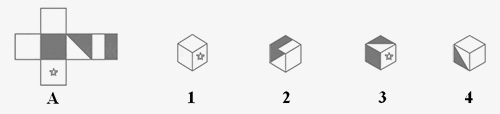A. 1
B. 2
C. 3
D. 4
Q.2. In the given figure what will be on the surface opposite of F?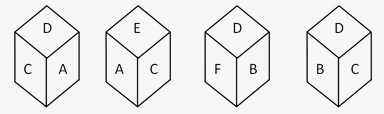A. A
B. B
C. C
D. D
Q.3. Which symbol will be opposite to the face containing the symbol ?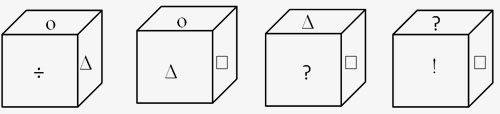A. !
B. Δ
C. ?
D. ÷
Q.4. Two of the positions of dice are represented in the figure below. Which of the faces will be opposite to the face with 5?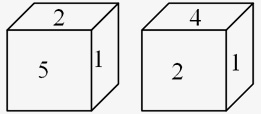A. 1
B. 2
C. 3
D. None of the above
Q.5. Which of the following numbers will we find on the face opposite to the face containing the number 2?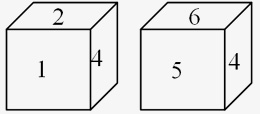A. 5
B. 4
C. 6
D. 1 or 4
Q.6. Two different positions of an unbiased dice are shown in the figure below. Which digit should appear on the face opposite to the face with the digit 3?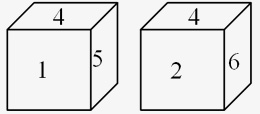A. 2 or 5
B. 6
C. 2
D. 3
Q.7. Which face is opposite to the face which contains 3?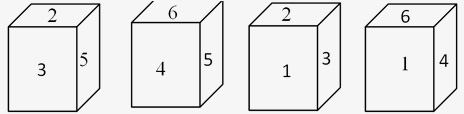A. 1
B. 5
C. 4
D. 6
Directions for Questions (8-10): The sheet of paper shown in the figure (A) given on the leftmost side, in each question, is folded to form a box. Choose from amongst the alternatives (1), (2), (3) and (4), the boxes that are similar to the box that will be formed.
Q8. Which option has boxes that are similar to the box made from the given sheet of paper (A).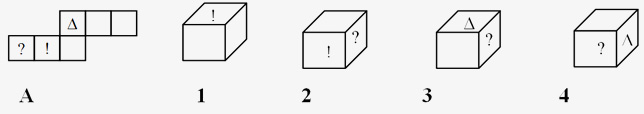A. 2 & 3 only
B. 1,2 & 3 only
C. 1,2,3 & 4
D. 1 only
Q9. Which option has boxes that are similar to the box made from the given sheet of paper(X).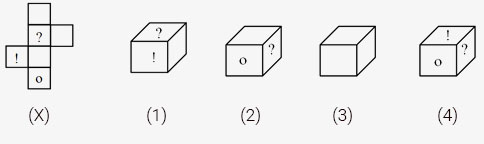A. 1, 3 and 4 only
B. 1 and 3 only
C. 1 only
D. 1, 2, 3 and 4
Q10. Which option has boxes that are similar to the box made from the given sheet of paper(X)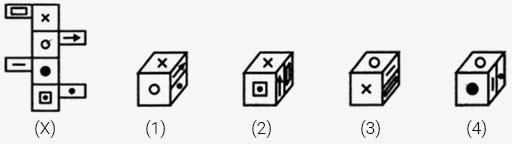A. 1, 2, 3 and 4
B. 1 and 2 only
C. 3 and 4 only
D. 1 and 3 only